﻿ On Extended Normal Inverse Gaussian Distribution: Theory, Methodology, Properties and ApplicationsPublications are Open
Access in this journal
Article Versions
Export Article
• Normal Style
• MLA Style
• APA Style
• Chicago Style
Research Article
Open Access Peer-reviewed

### On Extended Normal Inverse Gaussian Distribution: Theory, Methodology, Properties and Applications

Bachioua Lahcene
American Journal of Applied Mathematics and Statistics. 2019, 7(6), 224-230. DOI: 10.12691/ajams-7-6-4
Received October 15, 2019; Revised November 25, 2019; Accepted December 10, 2019

### Abstract

In this article, the Normal Inverse Gaussian Distribution model (NIGDM) is extended to a new Extended Normal Inverse Gaussian Distribution (ENIGDM) and its derivate models find many applications. The author proposes a new model ENIGDM, which generalizes the models of normal inverse Gaussian distribution. This class of ENIGDM is to approximate an unknown risk-neutral density. The paper discusses different properties of the ENIGDM. In particular, the applicability of this new general model with five parameters is well justified by more results which represent mixtures of inverse Gaussian distributions. Then a discussion is begun of the potential of the normal inverse Gaussian distribution and Lévy’s process for modeling and analyzing statistical data, with a particular reference to extensive sets of observations and applications in wide varieties.

### 1. History and Definition

The model of inverse Gaussian distribution is the inverse of one continuous normal distribution widely used in complex applications. This type of distribution was first derived by Schrödinger in 1915 during his study of the first time of the Brownian movement, which is addressed in the article 1. This distribution was proposed by Étienne Halphen 2. The inverse Gaussian name proposed by Tweedie was adopted in 1945 3.

The models of inverse normal distribution represent an exponentially composite model that has only one pattern and has a long tail to the right. This type is one of the distributions implemented in the generalized linear model tracking procedure 4. This distribution is used to track data with positive deviation, as well as a variety of diverse applications in economics and business, survival analysis, finance, medicine, and even in labor dispute resolution 5. Convolution of the inverse normal distribution model (a Wald distribution) and exponent (an ex-Wald distribution) is used as a model for response times in psychology 6.

The normal inverse Gaussian distribution model was first studied in a study aimed at tracking Brownian motion in physics in 1956. M.C.K. Tweedie first used it as Inverse Gaussian because there is an inverse relationship that expresses the time required to cover the distance of the unit and the distance covered at the time of the approved unit 7.

After the positive results obtained by the research, the model was adopted by Tweedie in 1957 and became known by the name, and then was determined and studied for some of its statistical characteristics. Due to the impressive results he has shown in practical applications, more articles have been devoted to this distribution among the continuous probability distributions that reflect various natural events, and have many practical applications in the field of probability and statistics branches which helped to show many statistical uses derived from the fact that errors Randomization often follows normal distribution 7.

Blaesild observed the reverse Gaussian distribution model in 1977 as a subclass of the generalized hyperbolic distribution discovered by Ole Barndorff-Nielsen 8. It is widely used in geological statistics, statistical linguistics, finance etc. It was rediscovered and widely disseminated by Ole Barndorff-Nielsen 9, who called it the normalized generalized reverse distribution model. Therefore, they are suitable for modeling phenomena where there is a higher probability of obtaining very high values compared to normal distribution 1.

The generalized general Gaussian distribution model is the distribution of a newly flexible closed shape that can be applied as a model for heavy-tailed operations. Also it is known as the Sichel distribution, after this, H. Barndorff-Nielsen and Halgreen proved that Gaussian inverse generalized distribution is indivisible and shows complete and expanded results 10. Its statistical characteristics are discussed further in several specialized research articles 11, 12.

Previously, Wald re-derived this distribution in 1947 as the preferred distribution showing the exact shape of a small sample in the sequential probability ratio test 3.

The reverse Gaussian normal distribution model is one of the commonly used distributions for modeling data lifetime or reaction time data and following the resulting improvements. When we examine reliability, the choice of distribution depends on the mechanism of failure, and is particularly useful for modeling long-tail data which are positively skewed 13.

Interested readers can refer to the books of Johnson et al where he explains many of the reasons why distribution has shown its positive form 14, and they can refer to Seshadri 2 for more details. The normal reverse Gaussian distribution model, also known as the Wald distribution, is the ideal candidate for modeling correct deviant positive data due to the fact that inference theory and methodology are very similar to natural theory and methods 15.

### 2. Mathematical Description and Theory

Let X is a random variable representing the normal inverse Gaussian distribution model NIGDM (1) and has a probability density function (pdf) with a one parameter given by: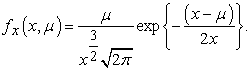(1)

We call this model the normal inverse Gaussian distribution model with one parameter defined by equation (1) and symbolized by the formula NIGDM (1). The expression of the model expressed in equation (1) has been expanded so that a new parameter has been added to become an identifier in terms of two parameters. The pdf form given by 16: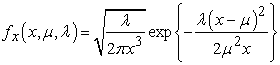(2)

Where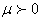is the mean and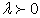is the shape parameter. We call this new form the normal inverse Gaussian distribution model and is symbolized by the formula NIGDM (2). The equation in formula (2) is generalized of equation (1) in case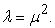In this form, the mean and variance of the distribution are equal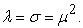A common special case of the above arises when the Brownian motion has no drift. In that case, parameter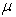tends to infinity, and the first passage time for fixed level α has pdf 15.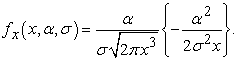(3)

The equation in formula (3) is generalized of equation (2) in case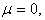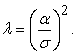This is a Lévy distribution with parameters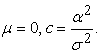The plot of the normal inverse Gaussian distribution model is a two-parameters and its pdf for various values of the parameters μ and λ is showed in Figure 1((a), (b)).

• Figure 1. ((a), (b)): Graph of fx(x, μ, λ) for various values of μ and λ

This is related to the canonical form of the two-parameters normal inverse Gaussian distribution model when written in it’s full form with scale parameter and location parameter by taking special cases where the parameter is used by the first model. The Figure 1 ((a), (b)) shows almost no impact of changing parameters and it is clear that the distribution has great flexibility in the representation of samples that show the mathematical models that follow this distribution 11.

Usually the mistake made by researchers is to assume that the inverse gamma tail will be cut in such a way that the image is beautiful. It turns out that when the volume λ is small, then it is possible to observe the presence of very large values, which are difficult to do without. Many cases have been demonstrated that by plotting graphs of random samples of various parameters settings can be oriented completing its information in a field close to the tail end of the curve. Some cases of the Gaussian inverse normal distribution model and the function of probability density values, and the cumulative distribution of different values of the parameters μ and λ are illustrated in Figure 2 ((a), (b)).

• Figure 2. ((a), (b)): Graph of fx(x, λ) and Fx(x, λ) for various values of μ and λ

The pdf of normal inverse Gaussian distribution model with three parameters ENIGDM (3) form given by 9;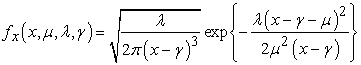(4)

Where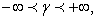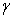is a threshold parameter ,is the mean andis the shape parameter. Hence, the ENIGDM (3) is an asymmetric distribution with high kurtosis for small values of the shape parameterbut gradually becomes symmetric with normal-like shape as the value of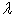increases.

The four-parameter extended Gaussian inverse natural distribution of ENIGDM (4) was first introduced by Barndorff-Nielsen (1997) as a normal medium-contrast mixture with Gaussian mixture distribution, which is studied in detail in the reference 7.

This generalization allows the elements of the ENIGDM (4) model identification to be fully demonstrated by four real-value parameters that have natural explanations that show great flexibility when representing the target data. After this model, the researcher can design a wider variety of pure and mixed distribution data. This model is often used in financial applications because of the weight of the distributions tail which shows the emergencies occurring in the tail-represented limbs. The pdf function of the normal reverse Gaussian distribution model with four ENIGDM (4) parameters is given by the following equation by;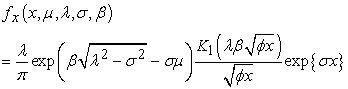(5)

Where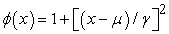and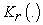denotes the modified Bessel function of the third kind of order r evaluated at x. A particularly important property is that the ENIGDM (4) is closed under convolution. Each parameter of the pdf plays its own role on approximating the curve to the data.

μ is the location parameter and determines the shift of the distribution

λ determines the tail heaviness (tail heaviness (real)).

σ determines the asymmetry of the distribution (scale parameter(real)).

β is the scale parameter and determines how spread out the distribution is.

The ENIGDM (4) model is a flexible, modern, closed-form distribution that can be applied as a model for heavy-tailed operations. These results encouraged researchers to look for broader models, and ENIGDM (4) of Barndorff-Nielsen was extended. The resulting model has a more flexible lag structure than the original model, and the density function of this pdf is referred to in the literature as Bessel modified type III. In 2013, Barndorff-Nielsen et al submitted one pdf of the Lévy distribution model with five parameters form by 8;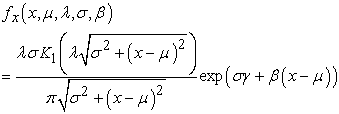(6)

Where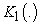denotes the modified Bessel function of the third kind of order 1 evaluated at x. During the generalized formula, normal inverse Gaussian distribution is defined as a variance-mean and seen to be a medium variation of the mixing distribution. The vocabulary of the distribution is required to be a homogeneous and integrated Lévy model of the random process. The process of determining the parameters of the inverse type Lévy contributes to tracking the decomposition of the random phenomenon 8.

In addition, the most important characteristic of the model is that the volatility and sequence of processes are derived. The ENIGDM (4) model is not included in the statistics toolbox because it expresses traces in the tail part, and because the model can also be considered as a specific phenomenon of emergency variability, which justifies the generalization and extension of the pdf function of ENIGDM (4) in terms of five key parameters.

### 3. New Extended Normal Inverse Gaussian Distribution

A review of some of these models includes the exponentiated Normal Inverse Gaussian distribution. In the last few years, new classes of distributions were proposed by extending the Normal Inverse Gaussian. The new model is named as new extended Normal Inverse Gaussian distribution. The pdf of normal inverse Gaussian distribution model with four parameters ENIGDM (5) distribution model with five parameters form by;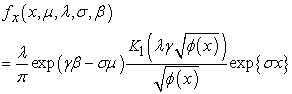(7)

Whereand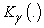denotes the modified Bessel function of the third kind of order r evaluated at x.

The suggested parameters for the modified model are five parameters;

μ is a location parameter.

λ is a tail heaviness parameter.

σ is a asymmetry scale parameter.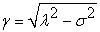is a threshold parameter.

β is a scale spread parameter.

The suggested gamma parameter is expressed as the square root )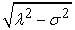) of the squares of λ and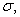which is known only when the parameter values are limited to the field in which the square root value is determined in the conventional form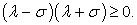The identification of the domain for each parameter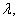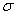and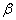allows the scope of its application and the sensing of forecasts of financial indicators and the stock exchange, which is proposed for future research.

In the scope of clarifying some aspects of the extension of the formula of the proposed model we review the specific case, and clarify the development of the threshold parameter formula in the new model presented in terms of pdf as follows: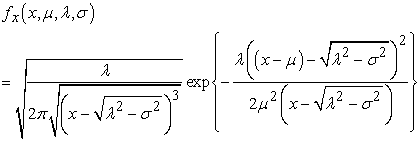(8)

We call this new form the extended normal inverse Gaussian distribution modified with three parameters and symbolized by the formula ENIGDM (3). The formula suggested in equation (8) is the same as the formula proposed in equation (4) with some modification of the threshold parameter.

In order to clarify this model expressed in terms of three parameters, we review the most basic properties. Then we study the model in the case of a mixture of models from this model proposed.

### 4. Properties of Normal Inverse Gaussian Distribution Model

The following proposition shows the important properties of the normal-inverse Gaussian distribution.

Property (1): The normal-inverse Gaussian distribution is a two-parameters family NIGDM (2). With natural parameters (-λ/2μ2) and (-λ/2), and natural statistics X and (1/X).

Proof: The result follows by using the transformation technique. The proof of this is simple and straightforward.

Property (2): Let X be a Random variable with normal-inverse Gaussian distribution of two parameters NIGDM(2). Then

1.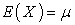2.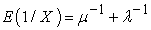3.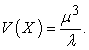4.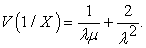Proof: By definition of the normal-inverse Gaussian distribution, the proof of this is simple and straightforward.

Property (3): Let X be a Random variable with normal-inverse Gaussian distribution of two parameters NIGDM (2). Then

1.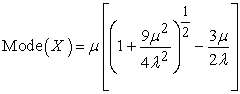2.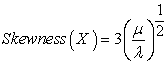3.Proof: By definition of the normal-inverse Gaussian distribution, the proof of this is simple and straightforward.

Property (4): Let be a Random variable with the normal-inverse Gaussian distribution of two parameters NIGDM (2). Then

1.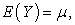2.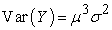Proof: The result follows by using the transformation technique. The result follows by changing the formula of parameters.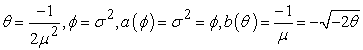By definition of the NIGDM (2) distribution, we have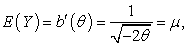and the variance of is obtained as followsProperty (5): Let be a Random variable with the normal-inverse Gaussian distribution of two parameters NIGDM (2)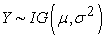(Inverse Gaussian distribution). Then the cumulative distribution function is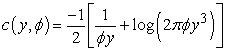Proof: The result follows by using the transformation technique. By definition of the normal-inverse Gaussian distribution, we have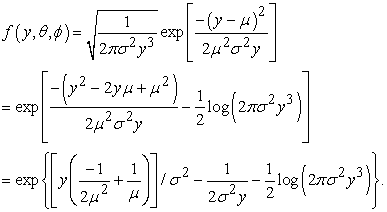For changing parameters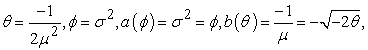Then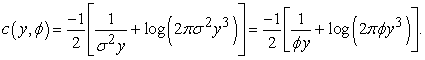Property(6): If and are independent random variables that are NIGDM (2) normal inverse Gaussian distributed with the same two values of the parameters α and β, but possible different values of the location and scale parameters μ11 and μ22 respectively, then X1+X2 is ENIGDM (4) extended normal inverse Gaussian distributed with four parameters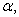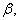μ12 and σ12.

Proof: The result represents the class of normal inverse Gaussian distributions which is closed under convolution in the following sense. The proof of this is simple and straightforward.

Property (7): Let X be a Random variable with extended normal-inverse Gaussian distribution of two parameters ENIGDM (5). Then

1.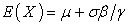2.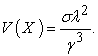3.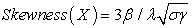4.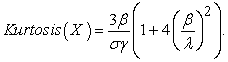Proof: By definition of the normal-inverse Gaussian distribution, the proof of this is simple and straightforward.

### 5. Mixtures of Normal Inverse Gaussian Distributions

The normal inverse Gaussian distribution model is defined as a variance-mean mixture of a normal distribution with the inverse Gaussian as the mixing distribution 11. Some extensions of the stochastic volatility type are constructed via an observation-driven approach to state space modeling. Interestingly, NIGDM (3) distribution is a versatile distribution in its own right and has been used in fatigue crack growth and reliability; see Birnbaum and Saunders 17.

We consider the filtering problem for a partially observable stochastic process, and the solution of nonlinear system taken by solution of difference equations, which provides a stochastic metallization for parameters of the normal inverse Gaussian distribution model.

Extended normal inverse Gaussian distribution modified with three parameters ENIGDM (3) is a right skewed distribution and it plays an important role in reliability analysis. Because of its exibility and several other interesting properties, it has always been a popular alternative to the recent modifications of extended Weibull family’s distributions 18, log-normal, gamma and other similar skewed distributions 17. Jorgensen, Seshadri and Whitmore 19 introduced a new distribution of a new NIGDM (3) which was presented as follows.

Suppose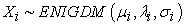and it has the pdf as follows: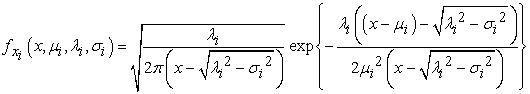Then consider the new random variable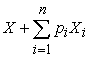such that the pdf as follows: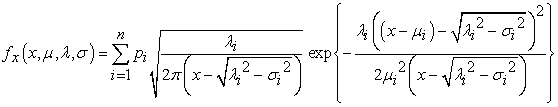Where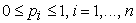for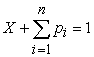and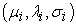is the parameters of the ENIGDM (3) of the i-th component. For n=2 suppose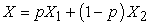is a mixture of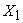and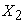and the probability density function (pdf) of X is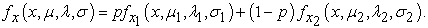ENIGDM (3) is a right skewed distribution and it plays an important role in reliability analysis because of its exibility and several other interesting properties. Due to these properties, considerable results have been made by aspects of this mixture of distributions.

It should be mentioned here that Kundu, Kannan and Balakrishnan 20 also studied independently the shape of the hazard function of the special case of ENIGDM (3) and provided some approximation of the change point of its hazard function.

The readers are referred to Gupta and Akman for simple cases related with this model 3. Therefore many key properties of the ENIGDM (3) model will be directly applicable here for different reliability properties of the ENIGDM (3). A statement of the normal-inverse Gaussian distribution was drawn as a mixture of two vehicles of the same normal-inverse Gaussian distribution as shown in Figure 3. ((a), (b)). It is clear that the mixture gives an extra charge to follow and explore the sub-characteristics of each distribution of the mixture, thus providing additional potential for applications.

• Figure 3. ((a), (b)): shows the probability density function of the normal-inverse Gaussian distribution mixture data in terms of three parameters μ, λ and σ

### 6. Applications and Conclusions

The studied references showed many special and basic applications of some models derived from the general model of the extended normal inverse Gaussian distribution. The normal inverse Gaussian distribution model has many similarities to standard Gaussian (normal) distributions, which lead to applications in inferential statistics. Thus, the most important applications are as follows:

Extended normal inverse Gaussian distribution model family are very interesting for applications. These areas of application imply a need for extending the inverse Gaussian Lévy process so as to accommodate certain, frequently observed, temporal dependence structures.

The Extended normal inverse Gaussian distribution model was first introduced in 9, 21 to model financial processes and has since then found many applications. It was introduced to signal processing in financial processes 22.

The Entropy characterization of the inverse Gaussian family and using it was to construct a goodness-of-fit test for ascertaining appropriateness of such models. Goodness-of-fit test for the inverse Gaussian distribution chance to study the new entropy estimation using simple random sampling techniques 11.

ENIGDM (3) obtains a superior fit compared to the Gaussian model when applied to spot price data from the oil and gas markets. Each responsibility is addressed to a particular basic case of the general extension formula in terms of three parameters. The main one in this case is the expression of the threshold parameter in terms of two parameters whose range can be determined for the value of the square root to take the real values.

### References

  Schrodinger E., (1915). "Zur Theorie Der Fall-und Steigversuche an Teilchen Mit Brownscher Bewegung", Physikalische Zeitschrift, Vol. (16), pp 289-295. In article  Seshadri, V., (1997). "Halphen's Laws". In Kotz, S.; Read, C. B.; Banks, D. L. Encyclopedia of Statistical Sciences, Update Volume 1. New York: Wiley. pp. 302-306. In article  Folks, J. L.; Chhikara, R. S., (1978). "The Inverse Gaussian Distribution and Its Statistical Application -A Review-", Journal of the Royal Statistical Society. Series B (Methodological), Vol. (40), No.(3): pp 263-289. In article View Article  Wald, A., (1947). "Sequential Analysis", Wiley, NY, USA. In article  Tweedie, M. C. K., (1956). "Some Statistical Properties of Inverse Gaussian Distributions", Virginia Journal of Science, Vol(7), pp160-165. In article  Seshadri V., (1992). "The Inverse Gaussian Distribution: A Case Study in Exponential Families", Oxford University Press, Oxford, UK. In article  Chhikara, Raj; Folks, Leroy., (1989). "The Inverse Gaussian Distribution: Theory, Methodology and Applications", New York: Marcel Dekker. In article  Barndorff-Nielsen. Ole E., (1997). "Normal Inverse Gaussian Distributions and Stochastic Volatility Modelling", Scandinavian Journal of Statistics, Vol. (24), No. (1): pp 1-13. In article View Article  Perreault, L.; Bobée, B.; Rasmussen, P. F., (1999). "Halphen Distribution System I: Mathematical and Statistical Properties", Journal of Hydrologic Engineering, Vo.(4), No.(3): pp189. In article View Article  Jørgensen, Bent., (1982). "Statistical Properties of the Generalized Inverse Gaussian Distribution", Lecture Notes in Statistics, No.(9), New York–Berlin: Springer-Verlag. In article View Article  Bachioua, Lahcene., (2018). "On Extended Normal Distribution Model with Application in Health Care", International Journal of Statistics in Medical Research, Vol.(07 ), No.(03), pp 1-11. In article  Balakrishnan N. and Chen W.W.S., (1997). "CRC Handbook of Tables for Order Statistics from Inverse Gaussian Distributions with Applications", CRC Press, Boca Raton, FL, USA. In article  Wald A., (1944). "On Cumulative Sums of Random Variables", Ann. Math. Stat, Vol. (15), No.(1944), pp. 283-296. In article View Article  Johnson N.L., S. Kotz, and Balakrishnan N., (1994)."Continuous Univariate Distributions", 2nd ed., Vol.(1), John Wiley & Sons, New York. In article  Lili Tian., (2006)."Testing Equality of Inverse Gaussian Means Under Heterogeneity, Based on Generalized Test Variable", journal Computational Statistics & Data Analysis, Vol.(51), No.(2), pp 1156-1162. In article View Article  Barndorff-Nielsen. Ole E., Mikosch, Thomas, Resnick, Sidney I., (2013). "Lévy Processes: Theory and Applications", Birkhäuser , Birkhäuser Science, Springer Nature, Global publisher. In article  Birnbaum, Z.W. and Saunders, S.C., (1969). "A New Family of Life Distributions", Journal of Applied Probability, Vol.(6): pp 319-327. In article View Article  Bachioua, Lahcene., (2018). "On Recent Modifications of Extended Weibull Families Distributions and Its Applications", Asian Journal of Fuzzy and Applied Mathematics, Vol. (06), No.(01), February, pp 1-11. In article  Jorgensen, B., Seshadri, V. and Whitmore, G.A. (1991). "On The Mixture of the Transseger Gaussian Distribution With its Complementary Reciprocal", the Scandinavian Journal of Statistics, vol. (18): pp 77-89. In article  Kundu, D., Kannan, N., Balakrishnan, N., (2008)."On the Hazard Function of Birnbaum-Saunders Distribution and Associated Inference", Computational Statistics & Data Analysis, Vol.(52), pp 2692-2702. In article View Article  Patel M.N., (1998). "Progressively Censored Samples From Inverse Gaussian Distribution, Aligarh J. Statist. Vol. (17), No. (18), pp28-34. In article  Alfred Hanssen., Tor Arne., (2001). "The Normal Inverse Gaussian Distribution: a Versatile Model for Heavy-Tailed Stochastic Processes". Proceedings - ICASSP, IEEE International Conference on Acoustics, Speech and Signal Processing. In article View Article  Bergdorf-Nielsen, Ole., (1977). "Exponentially Decreasing Distributions for the Logarithm of Particle Size", Proceedings of the Royal Society of London. Series A, Mathematical and Physical Sciences. The Royal Society, Vol.(353), No.(1674): pp 401-409. In article View Article  Dimitris Karlis., (2002). "An EM Type Algorithm for Maximum Likelihood Estimation of the Normal-Inverse Gaussian Distribution", Statist Probab. Lett., Vol(57), No.(1): pp 43-52. In article View Article  Gupta, R.C. and Akman, O., (1995). "On the Reliability Studies of a Weighted Inverse Gaussian Model", Journal of Statistical Planning and Inference, Vol. (48): pp 69-83. In article View Article  Prasanta Basak & Balakrishnan.N., (2012)."Estimation for the Three-Parameters Inverse Gaussian Distribution Under Progressive Type-II Censoring", Journal of Statistical Computation and Simulation, Vol. (82), No.(1). In article View Article  Schwarz, W., (2001). "The Ex-Wald Distribution as a Descriptive Model of Response Times", Behavior Research Methods, Instruments, and Computers, Vol. (33), No.(4): pp 457-69. In article View Article  PubMedThis work is licensed under a Creative Commons Attribution 4.0 International License. To view a copy of this license, visit http://creativecommons.org/licenses/by/4.0/